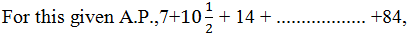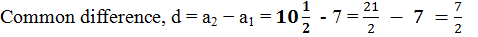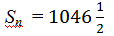Guru

# Find the sums given below

• 0

What is the best to solve the question of arithmetic progressions of exercise 5.3 of math. Please help me for solving this problem Find the sums given below

Share

1.First term, a = 7

nth term, an = 84Let 84 be the nth term of this A.P., then as per the nth term formula,

an = a(n-1)d

84 = 7+(n – 1)×7/2

77 = (n-1)×7/2

22 = n−1

n = 23

We know that, sum of n term is;

Sn = n/2 (a + l) , l = 84
Sn
= 23/2 (7+84)

Sn  = (23×91/2) = 2093/2• 0